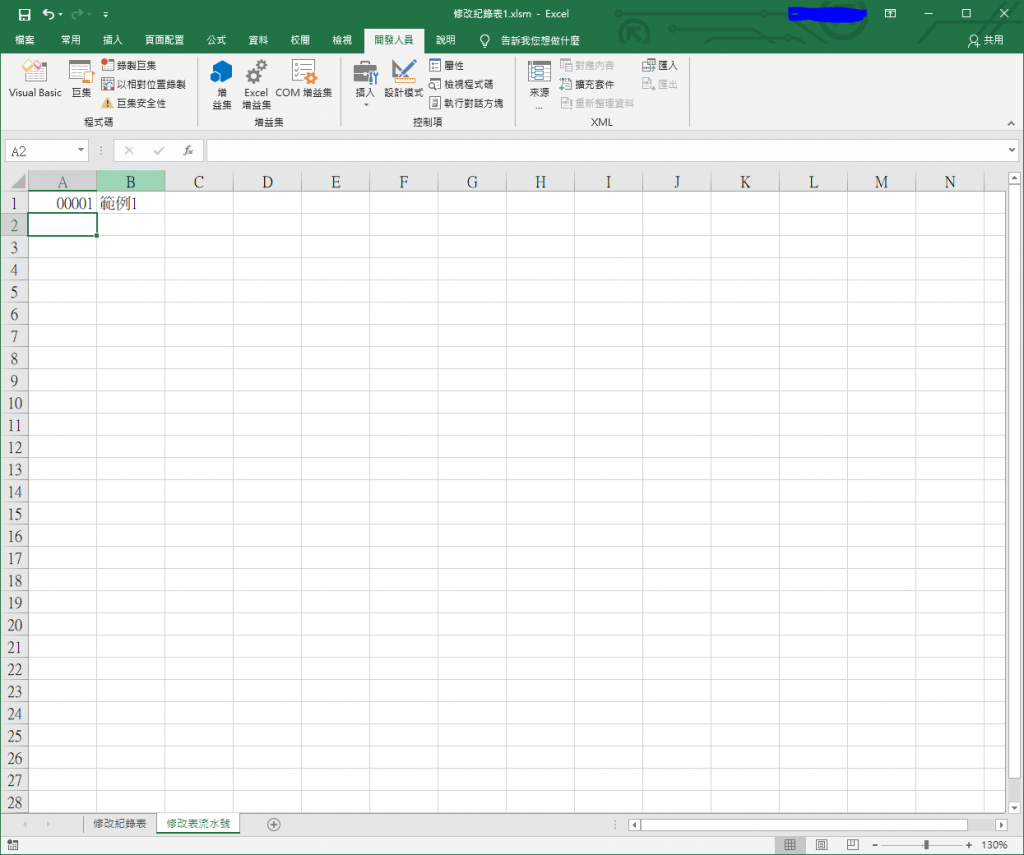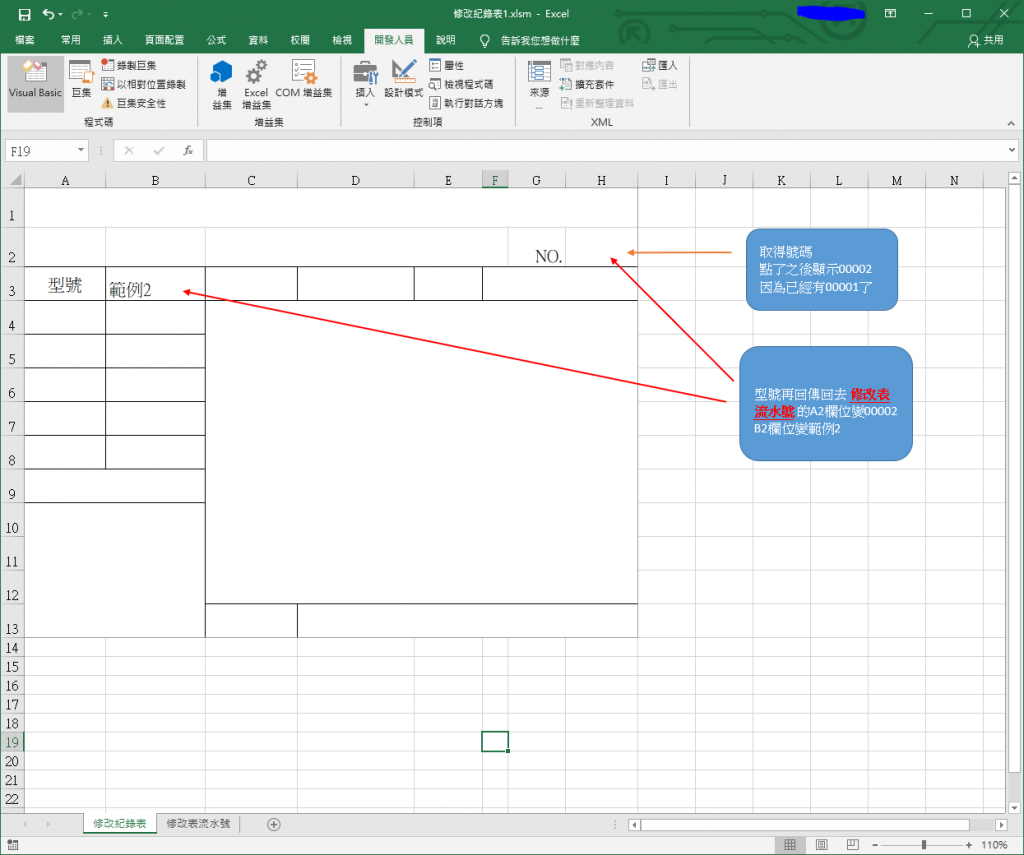#0

## 有沒有vba高手能幫我製作一下excel功能ccutmis iT邦高手 8 級 ‧ 2019-02-26 11:20:39 檢舉

### 1 個回答

0
``````Sub Main
Sheets("修改表流水號").Range("A1") = Sheets("修改表流水號").Range("A1") + 1
Sheets("修改表流水號").Range("B1") = "範例" & A1
Sheets("修改紀錄表").Range("H2") = A1
Sheets("修改紀錄表").Range("B3") = B1
End Sub
``````CalvinKuo iT邦大師 7 級 ‧ 2019-02-26 11:20:59 檢舉

ccutmis iT邦高手 8 級 ‧ 2019-02-26 11:22:33 檢舉

woody821119

``````Sub abc()

If 工作表1.Cells(1, 1) = "" Then
工作表2.Cells(1, 2).Value = 1
工作表1.Cells(1, 1).Value = 1
工作表1.Cells(1, 2) = 工作表2.Cells(1, 1).Value
End If
If 工作表2.Cells(1, 2) <> "" And 工作表2.Cells(2, 1) <> "" Then
For j = 2 To 工作表2.Cells(1, 1).End(xlDown).Row
If 工作表1.Cells(j, 1) = "" Then
For i = 1 To 工作表2.Cells(1, 1).End(xlDown).Row
If 工作表2.Cells(i, 2) = "" Then
工作表2.Cells(i, 2).Value = CInt(工作表1.Cells(j - 1, 1).Value) + 1
工作表1.Cells(j, 1) = 工作表2.Cells(i, 2).Value
工作表1.Cells(j, 2) = 工作表2.Cells(i, 1).Value
Exit For
End If
Next
End If
Next
End If
End Sub
``````

woody821119